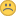5.2.1: DB upgrade write behind malloc()ed area:-(

XMLWordPrintable

Details

•Problem report
• Status: Closed
•Trivial
• Resolution: Duplicate
• 5.2.1
• None
• ARM 32 Ubuntu 14.04 (Odroid X2) - Zabbix compiled from source

Description

Steps to reproduce:

• I tried to upgrade my little home zabbix server from 5.0.4 to 5.2.1
• The DB upgrade progressed through DBpatch_5010043
• DBpatch_5010044 never completed.
• So I started to add debug prints and eventually, I found, that a malloc() did not succeed• So I tried to do a valgrind run and found two behind-by-1 writes. The lines will be a bit offset because of my added logging lines.
==14329== ERROR SUMMARY: 0 errors from 0 contexts (suppressed: 6 from 3)
==14330== Invalid write of size 1
==14330==    by 0x2CDD9: MAIN_ZABBIX_ENTRY (server.c:1183)
==14330==    by 0xE80ED: daemon_start (daemon.c:398)
==14330==    by 0x2C2BF: main (server.c:975)
==14330==  Address 0x56532ec is 0 bytes after a block of size 100 alloc'd
==14330==    at 0x482E358: malloc (vg_replace_malloc.c:296)
==14330==    by 0x4830D3F: realloc (vg_replace_malloc.c:692)
==14330==    by 0xF31C5: zbx_realloc2 (misc.c:660)
==14330==    by 0x2CDD9: MAIN_ZABBIX_ENTRY (server.c:1183)
==14330==    by 0xE80ED: daemon_start (daemon.c:398)
==14330==    by 0x2C2BF: main (server.c:975)
==14330==
==14330== Invalid write of size 1
==14330==    by 0x2CDD9: MAIN_ZABBIX_ENTRY (server.c:1183)
==14330==    by 0xE80ED: daemon_start (daemon.c:398)
==14330==    by 0x2C2BF: main (server.c:975)
==14330==  Address 0x56533cc is 0 bytes after a block of size 100 alloc'd
==14330==    at 0x482E358: malloc (vg_replace_malloc.c:296)
==14330==    by 0x4830D3F: realloc (vg_replace_malloc.c:692)
==14330==    by 0xF31C5: zbx_realloc2 (misc.c:660)
==14330==    by 0x2CDD9: MAIN_ZABBIX_ENTRY (server.c:1183)
==14330==    by 0xE80ED: daemon_start (daemon.c:398)
==14330==    by 0x2C2BF: main (server.c:975)

• The problematic loop:
for (i = 0; i < dim_x->values_num; i++)
{
if (POS_EMPTY != dim_x->values[i])
offsets_x->values[i + 1] = i == 0 ? dim_x->values[i] : offsets_x->values[i] + dim_x->values[i];
if (POS_EMPTY != dim_y->values[i])
offsets_y->values[i + 1] = i == 0 ? dim_y->values[i] : offsets_y->values[i] + dim_y->values[i];
}

• My quick-hack to avoid the problem (including one of the added prints):
@@ -661,21 +661,22 @@

static void    lw_array_debug(char *pfx, zbx_vector_char_t *v)
{
-       zabbix_log(LOG_LEVEL_TRACE, "%s: %s", pfx, lw_array_to_str(v));
+       // zabbix_log(LOG_LEVEL_TRACE, "%s: %s", pfx, lw_array_to_str(v));
+       zabbix_log(LOG_LEVEL_WARNING, "%s: %s", pfx, lw_array_to_str(v));
}

static zbx_vector_char_t       *lw_array_create(void)
{
zbx_vector_char_t       *v;
-       static char             fill[SCREEN_MAX_ROWS];
+       static char             fill[SCREEN_MAX_ROWS + 1];

if (0 == fill)
-               memset(fill, POS_EMPTY, SCREEN_MAX_ROWS);
+               memset(fill, POS_EMPTY, SCREEN_MAX_ROWS + 1);

v = (zbx_vector_char_t *)malloc(sizeof(zbx_vector_char_t));

zbx_vector_char_create(v);
-       zbx_vector_char_append_array(v, fill, SCREEN_MAX_ROWS);
+       zbx_vector_char_append_array(v, fill, SCREEN_MAX_ROWS + 1);

return v;
}

I hope, this can be checked and potentially fixed?!

Thanks,
– Marco

PeopleZabbix Development TeamMarco Walther